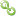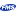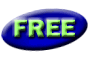Book RecommendationsLinks to Related SitesMy FMS

Thank you! Thank you! I just finished reading this document, which was part of a link in the recent Buzz newsletter. I have printed it for others to read, especially those skeptical on the powers of Access and its capabilities.

Darren D.# Use caution when Using CONVERT() with CASE or IF functions in Transact SQL (T-SQL)

### Provided by:  FMS Development Team

There are many articles available that cover the how-to's for using CONVERT() properly, however there is one that has very sparse coverage: Using CONVERT() with CASE or IF functions in Transact SQL (T-SQL) and what the expected results will be.

For a stored procedure I had a requirement that if the value was a number, it needed to show 2 decimal places, and if it was not a number, return the original value.  Of course it was all originally stored in the client table as nvarchar(50).

So I decided that using a Case statement was the most ideal.  However, I continually received error "Error converting data type nvarchar to numeric" using Convert inside of the Case statement.

Let's take the following example table and fields and values:

 IDField(int) ValueField(nvarchar(50)) 1 300 2 Test

If you try to convert the values to a Decimal datatype in a Case (or If) statement, all of the values returned will be expected to be Decimal datatype:

Select IDField, ValueField,
Case When IsNumeric(ValueField)<>0 THEN
CONVERT(decimal (10,2),ValueField)
ELSE
ValueField
End as ConvertedValue
From Table1

This fails with: "Error converting data type nvarchar to numeric."

Using the same example table, but ensuring both values are NOT numeric (and cannot be converted to a number), the above example still fails with the same error:

 IDField(int) ValueField(nvarchar(50) 1 Test 2 Test

So why does it fail with both of these?  I mean, the Convert line of code isn’t even being hit in the second example, right?

The first thoughts are generally one of the following "Since the first value evaluated is numeric, it is converted to decimal, and all other data is expected to be a decimal as well" OR "If SQL Server is able to convert ANY of the values to the specified type, then all values are expected to be of the converted type".  However, that's not correct (although the second is close)!

The real problem is that if you choose to Convert the values anywhere within the Case statement, the datatype you are converting the values to is the expected type of ALL the values regardless of if they are of that type or not.  Further, even if NONE of the values can actually be converted (even if the Convert line of code never executes), ALL of the values are still expected to be of the type specified by the Convert function!

So, how do you ensure that all of the datatypes returned are the same?  Well, if you don’t mind leaving the values as nvarchar, then there is a pseudo solution.  Simply ensure that your conversion to the datatype you want (decimal in our example), is converted back to the original field datatype again (nvarchar in our example):

Select IDField, ValueField,
Case When IsNumeric(ValueField)<>0 THEN
--convert it to the table's original field type nvarchar(50)
CONVERT(nvarchar(50),CONVERT(decimal(10,2),ValueField))
ELSE
ValueField
End as ConvertedValue
From Table1

Simply be cautious when converting datatypes, even if none of the values are in the desired format you want to convert to.  Always be sure to convert the values back to the original datatype to ensure you do not return errors like this one.